Navigate back to the homepage

# Parallel Mandelbrot Set Using Golang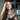Gisela Difini
June 17th, 2021 · 2 min read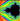This post explains how to generate a Mandelbrot set in parallel using Golang `goroutines`.

Source code here: https://github.com/GiselaMD/parallel-mandelbrot-go

## Mandelbrot Set

For those that are interest in what’s a Mandelbrot set, check https://en.wikipedia.org/wiki/Mandelbrot_set

The set formula is based on the position of `x` and `y` coordinates:

`.css-1chxjt6{position:absolute;right:22px;top:24px;padding:8px 12px 7px;border-radius:5px;color:#6f7177;-webkit-transition:background 0.3s ease;transition:background 0.3s ease;}.css-1chxjt6:hover{background:rgba(255,255,255,0.07);}.css-1chxjt6[data-a11y="true"]:focus::after{content:"";position:absolute;left:-2%;top:-2%;width:104%;height:104%;border:2px solid var(--theme-ui-colors-accent,#6166DC);border-radius:5px;background:rgba(255,255,255,0.01);}@media (max-width:45.9375em){.css-1chxjt6{display:none;}}1x = x*x - y*y + a2y = 2*x*y + b`

We also check if `x*x + y*y > 4` to set the color.

But instead of going into math details, I would like to explain how we can use `goroutines` to render that Mandelbrot set on the screen.

## Getting into the code

This program is based on 4 main values that are going to impact the performance and resolution of the Mandelbrot set.

`1maxIter = 10002samples = 20034numBlocks  = 645numThreads = 16`
• `maxIter` defines how many times the Mandelbrot formula will be calculated, resulting on `x` and `y` values.
• `samples` is the number of interactions that generates RGB color values.
• `numBlocks` is in how many pieces do you want to divide the image.
• `numThreads` is the number of `goroutines` that will be created.

To render the result on the screen I’ve used the Pixel library (github.com/faiface/pixel). On the main function we have something like this:

`1func main() {2    pixelgl.Run(run)3}`

Calling `pixelgl.Run` puts PixelGL in control of the main function and there’s no way for us to run any code in the main function anymore. That’s why we need to pass another function inside `pixelgl.Run`, which is the `run` function.

`1func run() {2    log.Println("Initial processing...")3    pixelCount = 04    img = image.NewRGBA(image.Rect(0, 0, imgWidth, imgHeight))5    cfg := pixelgl.WindowConfig{6        Title:  "Parallel Mandelbrot in Go",7        Bounds: pixel.R(0, 0, imgWidth, imgHeight),8        VSync:  true,9    }1011    win, err := pixelgl.NewWindow(cfg)12    if err != nil {13        panic(err)14    }15    log.Println("Rendering...")16    start := time.Now()17    workBuffer := make(chan WorkItem, numBlocks)18    threadBuffer := make(chan bool, numThreads)19    drawBuffer := make(chan Pix, pixelTotal)2021    workBufferInit(workBuffer)22    go workersInit(drawBuffer, workBuffer, threadBuffer)23    go drawThread(drawBuffer, win)2425    for !win.Closed() {26        pic := pixel.PictureDataFromImage(img)27        sprite := pixel.NewSprite(pic, pic.Bounds())28        sprite.Draw(win, pixel.IM.Moved(win.Bounds().Center()))29        win.Update()3031        if showProgress {32            fmt.Printf("\r%d/%d (%d%%)", pixelCount, pixelTotal, int(100*(float64(pixelCount)/float64(pixelTotal))))33        }3435        if pixelCount == pixelTotal {36            end := time.Now()37            fmt.Println("\nFinished with time = ", end.Sub(start))38            pixelCount++3940            if closeOnEnd {41                break42            }43        }44    }45}`

The `run` function is responsible for initialising and updating the window as well as creating the channels that will be used for our `goroutines`.

The `workBuffer` is the channel responsible for adding the information of each block (based on `numBlocks`). Inside the `workBufferInit`, the initial and final `x` and `y` values are sent to the channel so that each `goroutines` that gets that piece of the image to work on can calculate the color without needing to know the global data, only what’s the range of `x` and `y` of that block.

`1func workBufferInit(workBuffer chan WorkItem) {2    var sqrt = int(math.Sqrt(numBlocks))34    for i := sqrt - 1; i >= 0; i-- {5        for j := 0; j < sqrt; j++ {6            workBuffer <- WorkItem{7                initialX: i * (imgWidth / sqrt),8                finalX:   (i + 1) * (imgWidth / sqrt),9                initialY: j * (imgHeight / sqrt),10                finalY:   (j + 1) * (imgHeight / sqrt),11            }12        }13    }14}`

The `threadBuffer` is responsible for creating `goroutines` based on the `numThreads` and controlling when a `goroutine` is done with its work so we can run another in its place. That logic inside `workersInit` `goroutine`.

`1func workersInit(drawBuffer chan Pix, workBuffer chan WorkItem, threadBuffer chan bool) {2    for i := 1; i <= numThreads; i++ {3        threadBuffer <- true4    }56    for range threadBuffer {7        workItem := <-workBuffer89        go workerThread(workItem, drawBuffer, threadBuffer)10    }11}`

For each `workItem` that we receive from the `workBuffer` (each block) we create a `goroutine` called `workerThread` to handle all the Mandelbrot set logic.

`1func workerThread(workItem WorkItem, drawBuffer chan Pix, threadBuffer chan bool) {2    for x := workItem.initialX; x < workItem.finalX; x++ {3        for y := workItem.initialY; y < workItem.finalY; y++ {4            var colorR, colorG, colorB int5            for k := 0; k < samples; k++ {6                a := height*ratio*((float64(x)+RandFloat64())/float64(imgWidth)) + posX7                b := height*((float64(y)+RandFloat64())/float64(imgHeight)) + posY8                c := pixelColor(mandelbrotIteraction(a, b, maxIter))9                colorR += int(c.R)10                colorG += int(c.G)11                colorB += int(c.B)12            }13            var cr, cg, cb uint814            cr = uint8(float64(colorR) / float64(samples))15            cg = uint8(float64(colorG) / float64(samples))16            cb = uint8(float64(colorB) / float64(samples))1718            drawBuffer <- Pix{19                x, y, cr, cg, cb,20            }2122        }23    }24    threadBuffer <- true25}`
`1func mandelbrotIteraction(a, b float64, maxIter int) (float64, int) {2    var x, y, xx, yy, xy float6434    for i := 0; i < maxIter; i++ {5        xx, yy, xy = x*x, y*y, x*y6        if xx+yy > 4 {7            return xx + yy, i8        }9        // xn+1 = x^2 - y^2 + a10        x = xx - yy + a11        // yn+1 = 2xy + b12        y = 2*xy + b13    }1415    return xx + yy, maxIter16}1718func pixelColor(r float64, iter int) color.RGBA {19    insideSet := color.RGBA{R: 0, G: 0, B: 0, A: 255}2021    // check if it's inside the set22    if r > 4 {23        // return hslToRGB(float64(0.70)-float64(iter)/3500*r, 1, 0.5)24        return hslToRGB(float64(iter)/100*r, 1, 0.5)25    }2627    return insideSet28}`

The `drawBuffer` is the channel that receives the values from the `goroutines` that are calculating the Mandelbrot set and once it receives data, the `drawThread` `goroutine` sets the pixel RGB value into the image and then the `run` function updates the window.

`1func drawThread(drawBuffer chan Pix, win *pixelgl.Window) {2    for i := range drawBuffer {3        img.SetRGBA(i.x, i.y, color.RGBA{R: i.cr, G: i.cg, B: i.cb, A: 255})4        pixelCount++5    }6}`

We also have some utils functions for generating random data and converting hsl and hue to RGB:

`1var randState = uint64(time.Now().UnixNano())23func RandUint64() uint64 {4    randState = ((randState ^ (randState << 13)) ^ (randState >> 7)) ^ (randState << 17)5    return randState6}78func RandFloat64() float64 {9    return float64(RandUint64() / 2) / (1 << 63)10}1112func hueToRGB(p, q, t float64) float64 {13    if t < 0 { t += 1 }14    if t > 1 { t -= 1 }15    switch {16    case t < 1.0 / 6.0:17        return p + (q - p) * 6 * t18    case t < 1.0 / 2.0:19        return q20    case t < 2.0 / 3.0:21        return p + (q - p) * (2.0 / 3.0 - t) * 622    default:23        return p24    }25}2627func hslToRGB(h, s, l float64) color.RGBA {28    var r, g, b float6429    if s == 0 {30        r, g, b = l, l, l31    } else {32        var q, p float6433        if l < 0.5 {34            q = l * (1 + s)35        } else {36            q = l + s - l * s37        }38        p = 2 * l - q39        r = hueToRGB(p, q, h + 1.0 / 3.0)40        g = hueToRGB(p, q, h)41        b = hueToRGB(p, q, h - 1.0 / 3.0)42    }43    return color.RGBA{ R: uint8(r * 255), G: uint8(g * 255), B: uint8(b * 255), A: 255 }44}`

Final result: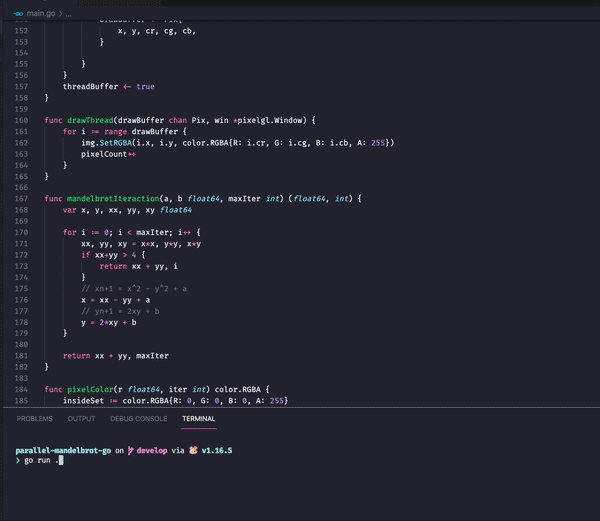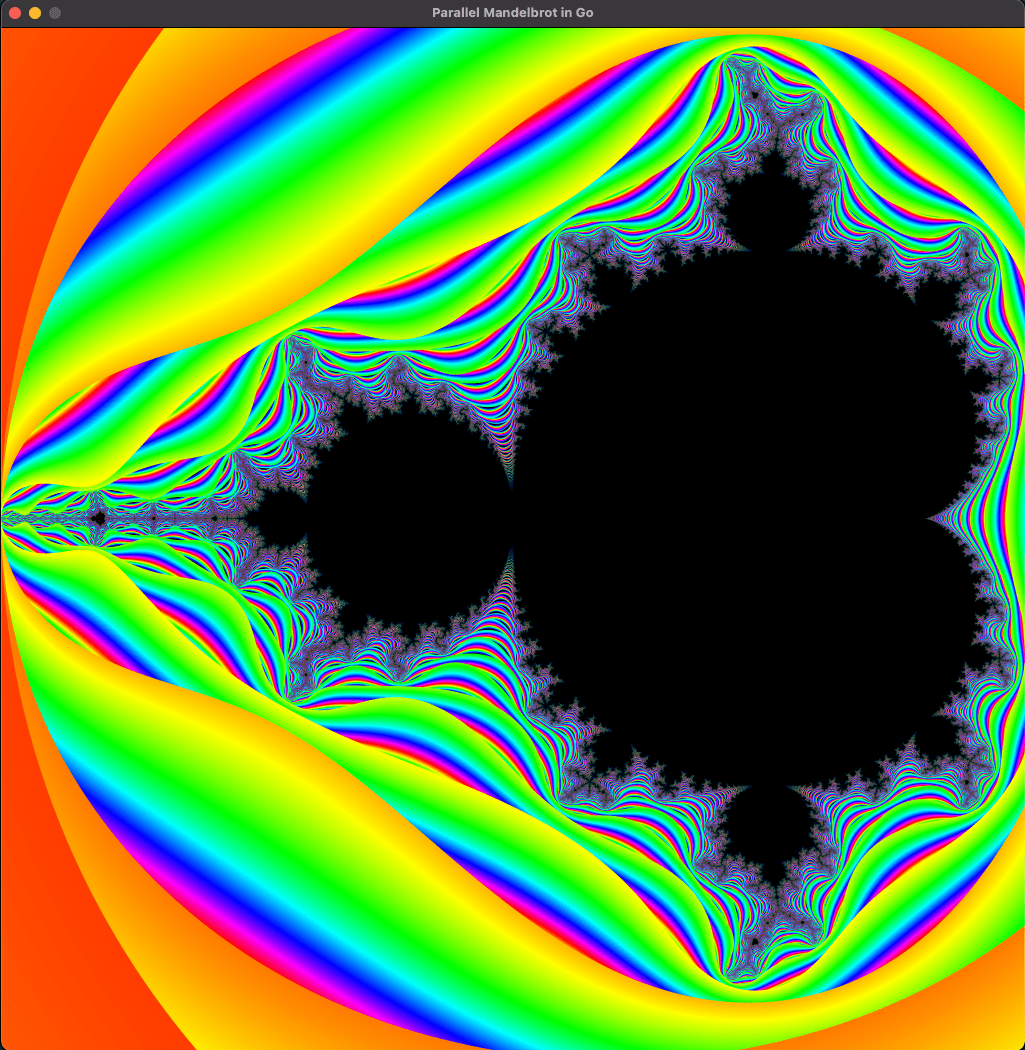That’s it for today!

Hope you enjoy it 😊

Source code here: https://github.com/GiselaMD/parallel-mandelbrot-go

### More articles from Gisela Miranda Difini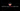### Creating a custom chart.js legend style

Hi! For those who don't know chart.js , it's a javascript chart library. Using a library for creating data visualization can be a little…

September 18th, 2020 · 1 min read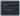### Creating a React Input component in typescript

🇺🇸 Here is a nice way to create a React Input component using typescript! Using an interface to declare your own prop types as well as…

August 9th, 2020 · 1 min read